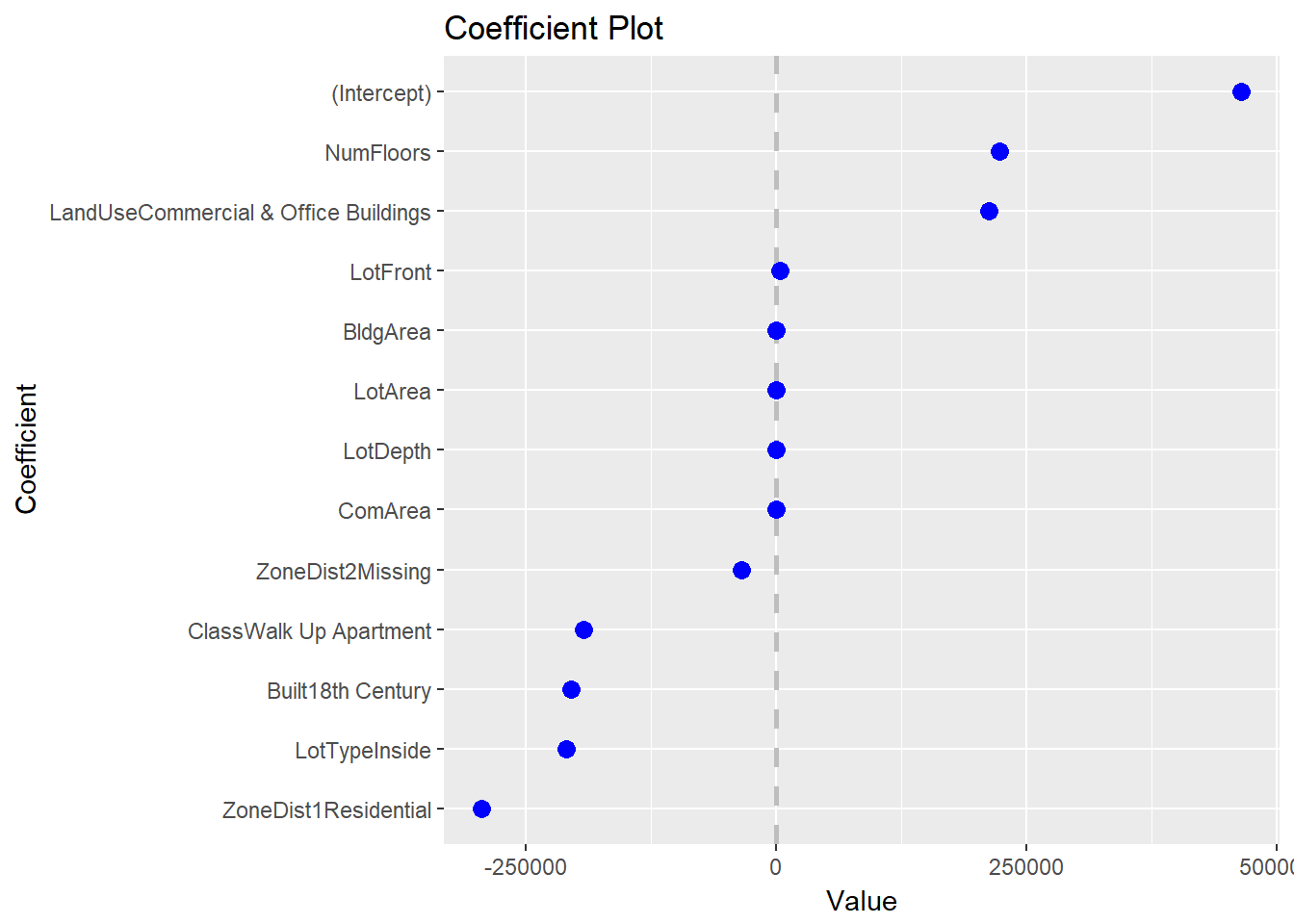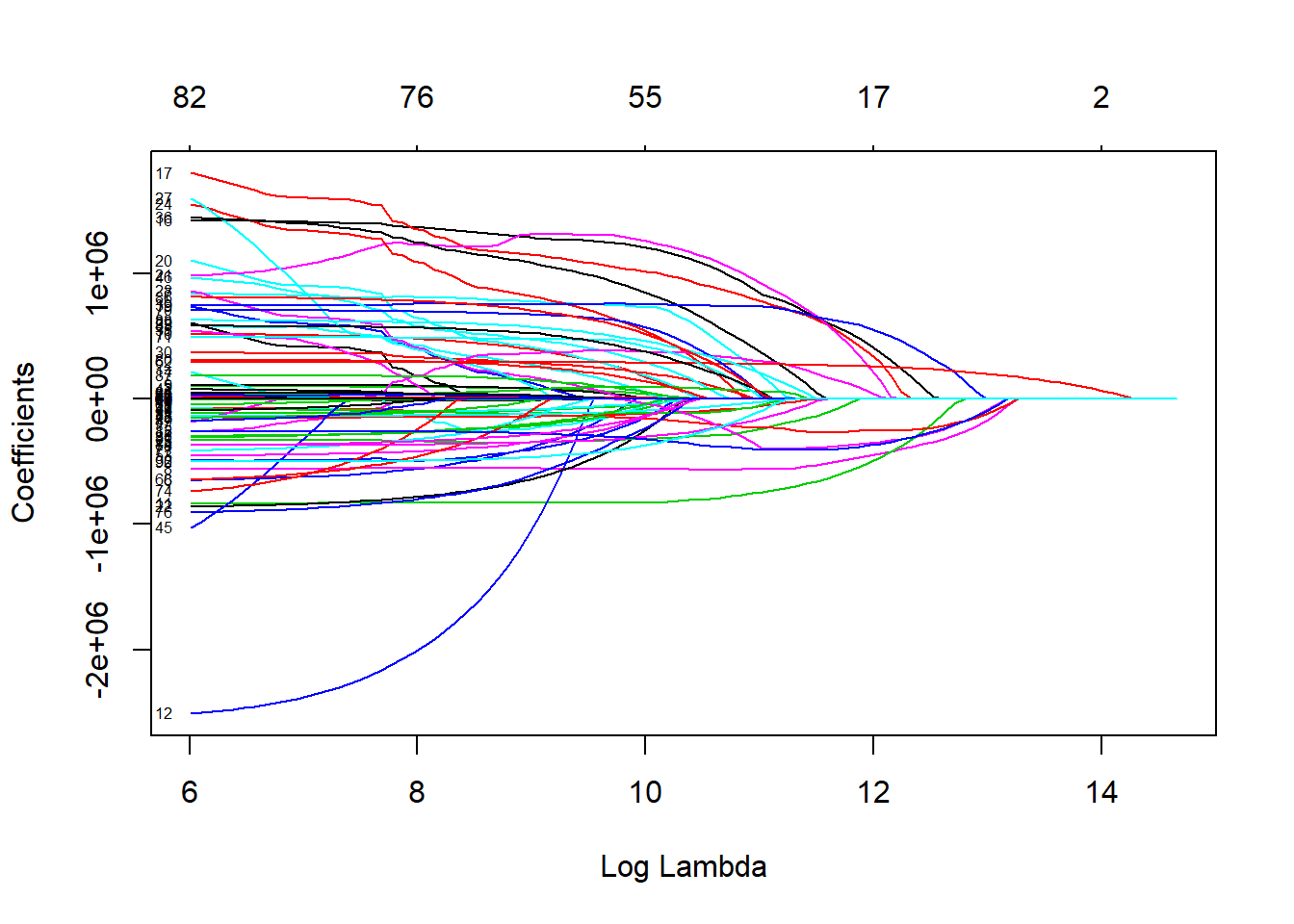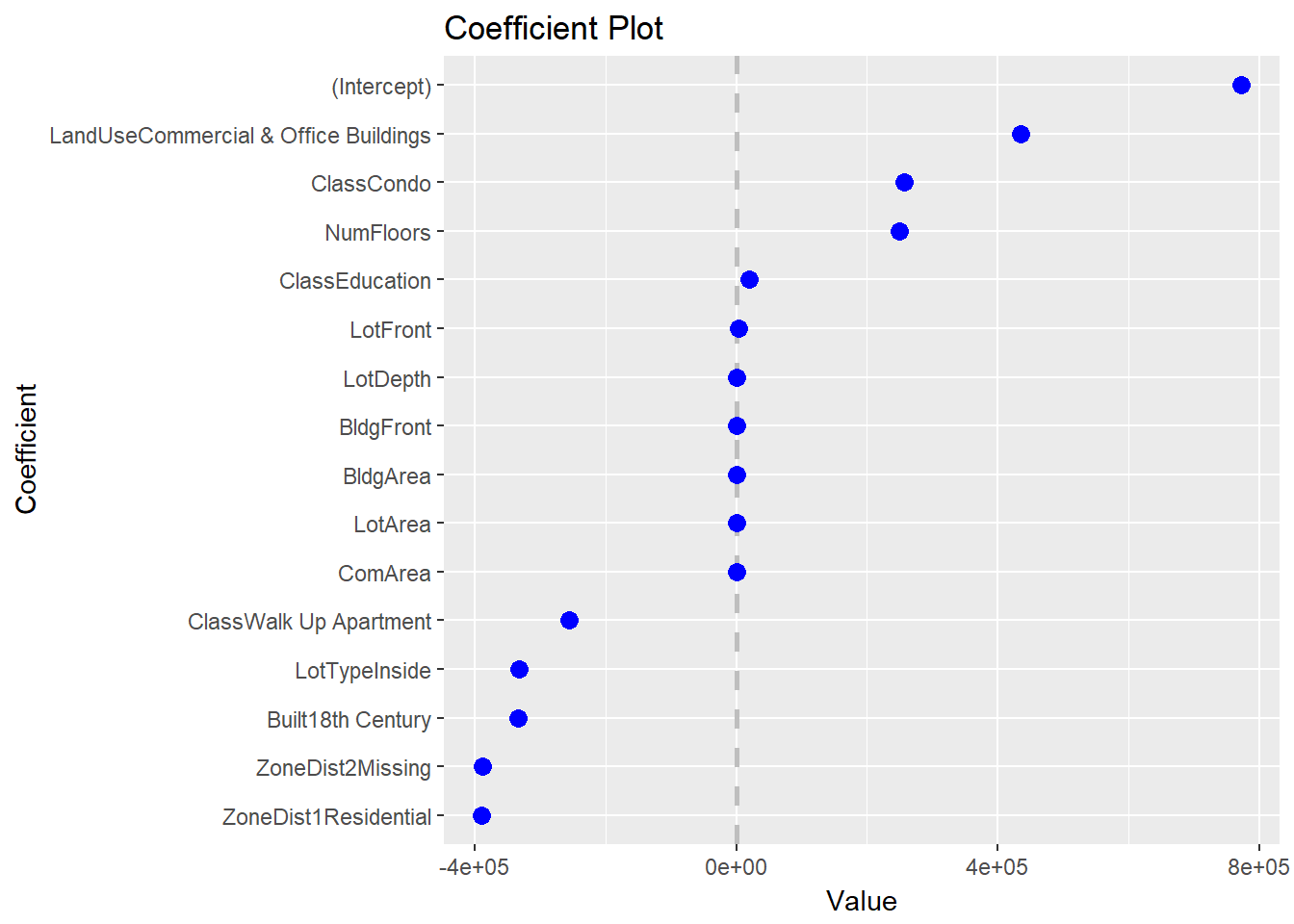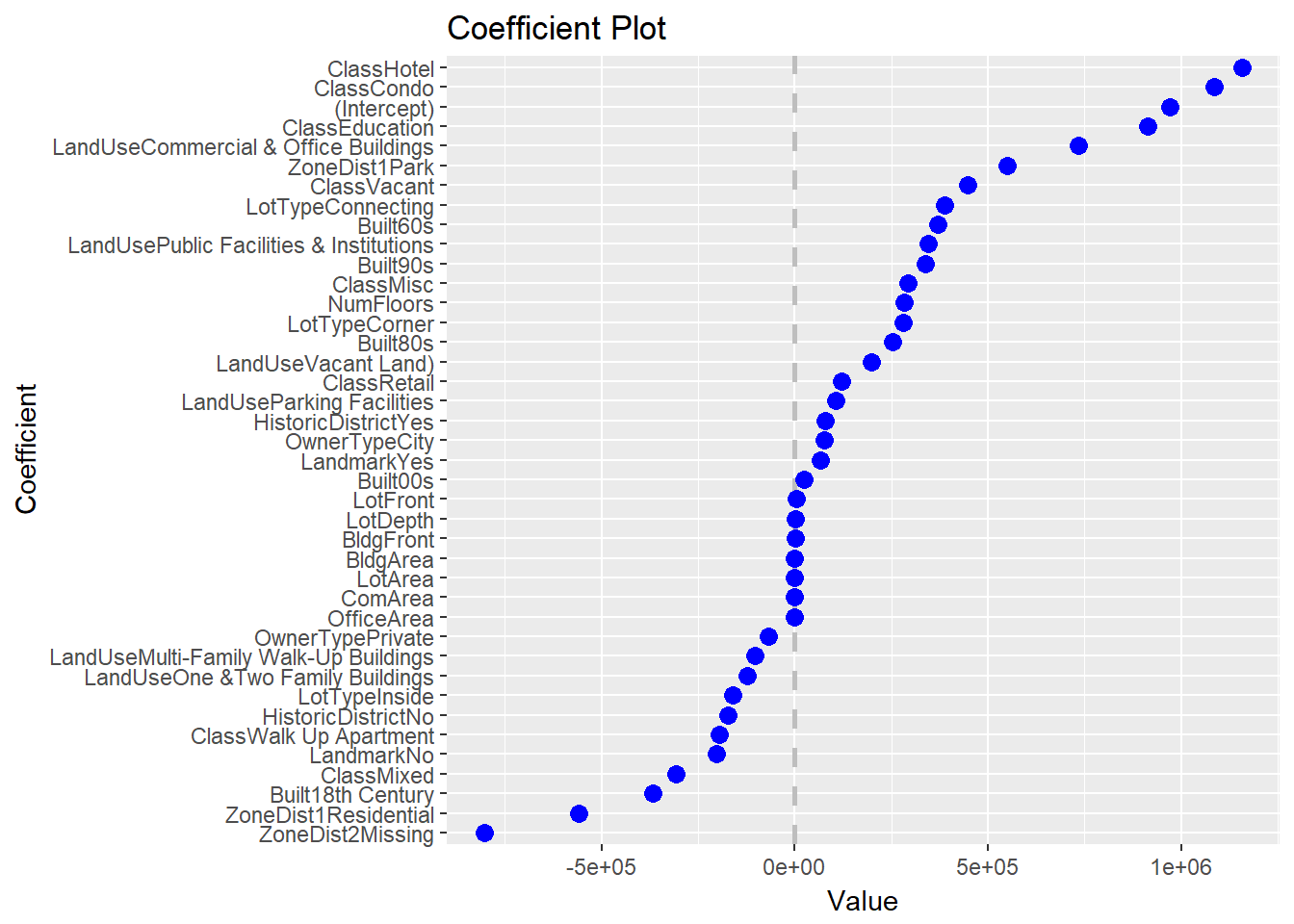I’m a big fan of the Elastic Net for variable selection and shrinkage and have given numerous talks about it and its implementation, `glmnet`. In fact, I will even have a DataCamp course about glmnet coming out soon.

As a side note, I used to pronounce it g-l-m-net but after having lunch with one of its creators, Trevor Hastie, I learn it is pronounced glimnet.

`coefplot` has long supported `glmnet` via a standard coefficient plot but I recently added some functionality, so let’s take a look. As we go through this, please pardon the `htmlwidgets` in iframes.

First, we load packages. I am now fond of using the following syntax for loading the packages we will be using.

``````# list the packages that we load
# alphabetically for reproducibility
packages <- c('coefplot', 'DT', 'glmnet')
# call library on each package
purrr::walk(packages, library, character.only=TRUE)

# some packages we will reference without actually loading
# they are listed here for complete documentation
packagesColon <- c('dplyr', 'knitr', 'magrittr', 'purrr', 'tibble', 'useful')``````

The versions can then be displayed in a table.

``````versions <- c(packages, packagesColon) %>%
purrr::map(packageVersion) %>%
purrr::map_chr(as.character)
packageDF <- tibble::data_frame(Package=c(packages, packagesColon), Version=versions) %>%
dplyr::arrange(Package)
knitr::kable(packageDF)``````
Package Version
coefplot 1.2.5.1
dplyr 0.7.4
DT 0.2
glmnet 2.0.13
knitr 1.18
magrittr 1.5
purrr 0.2.4
tibble 1.4.1
useful 1.2.3

First, we read some data. The data are available at http://www.jaredlander.com/data/manhattan_Train.rds with the CSV version at data.world.

``manTrain <- readRDS(url('http://www.jaredlander.com/data/manhattan_Train.rds'))``

The data are about New York City land value and have many columns. A sample of the data follows.

``````datatable(manTrain %>% dplyr::sample_n(size=100), elementId='DataSampled',
rownames=FALSE,
extensions=c('FixedHeader', 'Scroller'),
options=list(
scroller=TRUE,
scrollY=300
))``````

In order to use `glmnet` we need to convert our `tbl` into an X (predictor) `matrix` and a Y (response) `vector`. Since we don’t have to worry about multicolinearity with `glmnet` we do not want to drop the baselines of `factor`s. We also take advantage of sparse matrices since that reduces memory usage and compute, even though this dataset is not that large.

In order to build the `matrix` ad `vector` we need a `formula`. This could be built programmatically, but we can just build it ourselves. The response is `TotalValue`.

``````valueFormula <- TotalValue ~ FireService + ZoneDist1 + ZoneDist2 +
Class + LandUse + OwnerType + LotArea + BldgArea + ComArea + ResArea +
OfficeArea + RetailArea + NumBldgs + NumFloors + UnitsRes + UnitsTotal +
LotDepth + LotFront + BldgFront + LotType + HistoricDistrict + Built +
Landmark - 1``````

Notice the `- 1` means do not include an intercept since `glmnet` will do that for us.

``````manX <- useful::build.x(valueFormula, data=manTrain,
# do not drop the baselines of factors
contrasts=FALSE,
# use a sparse matrix
sparse=TRUE)

manY <- useful::build.y(valueFormula, data=manTrain)``````

We are now ready to fit a model.

``mod1 <- glmnet(x=manX, y=manY, family='gaussian')``

We can view a coefficient plot for a given value of `lambda` like this.

``coefplot(mod1, lambda=330500, sort='magnitude')``A common plot that is built into the `glmnet` package it the coefficient path.

``plot(mod1, xvar='lambda', label=TRUE)``This plot shows the path the coefficients take as `lambda` increases. They greater `lambda` is, the more the coefficients get shrunk toward zero. The problem is, it is hard to disambiguate the lines and the labels are not informative.

Fortunately, `coefplot` has a new function in Version 1.2.5 called `coefpath` for making this into an interactive plot using dygraphs.

``coefpath(mod1)``

While still busy this function provides so much more functionality. We can hover over lines, zoom in then pan around.

These functions also work with any value for `alpha` and for cross-validated models fit with `cv.glmnet`.

``mod2 <- cv.glmnet(x=manX, y=manY, family='gaussian', alpha=0.7, nfolds=5)``

We plot coefficient plots for both optimal `lambda`s.

``````# coefplot for the 1se error lambda
coefplot(mod2, lambda='lambda.1se', sort='magnitude')````````````# coefplot for the min error lambda
coefplot(mod2, lambda='lambda.min', sort='magnitude')``````The coefficient path is the same as before though the optimal `lambda`s are noted as dashed vertical lines.

``coefpath(mod2)``

While `coefplot` has long been able to plot coefficients from `glmnet` models, the new `coefpath` function goes a long way in helping visualize the paths the coefficients take as `lambda` changes.Jared Lander is the Chief Data Scientist of Lander Analytics a New York data science firm, Adjunct Professor at Columbia University, Organizer of the New York Open Statistical Programming meetup and the New York and Washington DC R Conferences and author of R for Everyone.## 2 thoughts on “Using coefplot with glmnet”

1.Hi Jared — I’m the guy who was in the front row at both your workshops at ODSC London last week. I’ve just bookmarked this to my employer’s corporate social media thang so my colleagues will understand what I’m talking about (and to help me find my way back to it, until I’ve bought your book). This will definitely be a big help in my work (TL;DR: emulators for complex physcis codes). Hope all continues well for you.

Reply
•I’m glad it has been so helpful! If you want any more help or onsite training we’re happy to help.

Reply